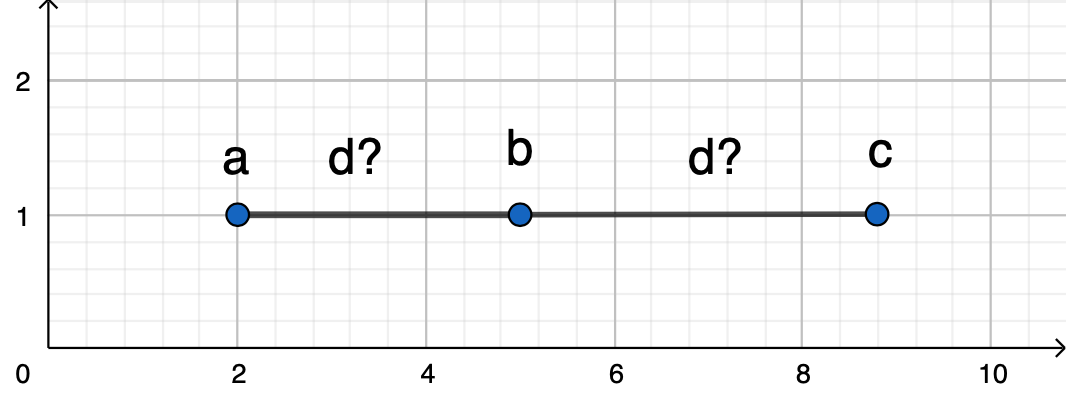# Which statement must be true?

5. If $a<b<c$ and $a<d<c$, which of the following statements must be true?

1. $a<c$
2. $b=d$
3. $b<d$

1. I only
2. II only
3. III only
4. I and II
5. I, II and III

$\text{We know that:} \begin{cases} a<b>c\\ a<d<c \end{cases}$

Which means both b and d lies between a and b as explained in following figure, but we don't know if b is equal to, greater or less than d.